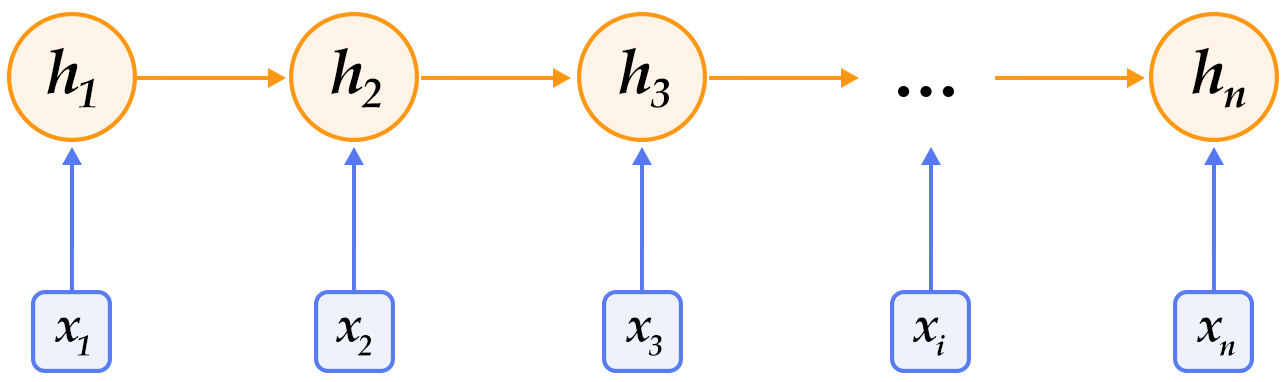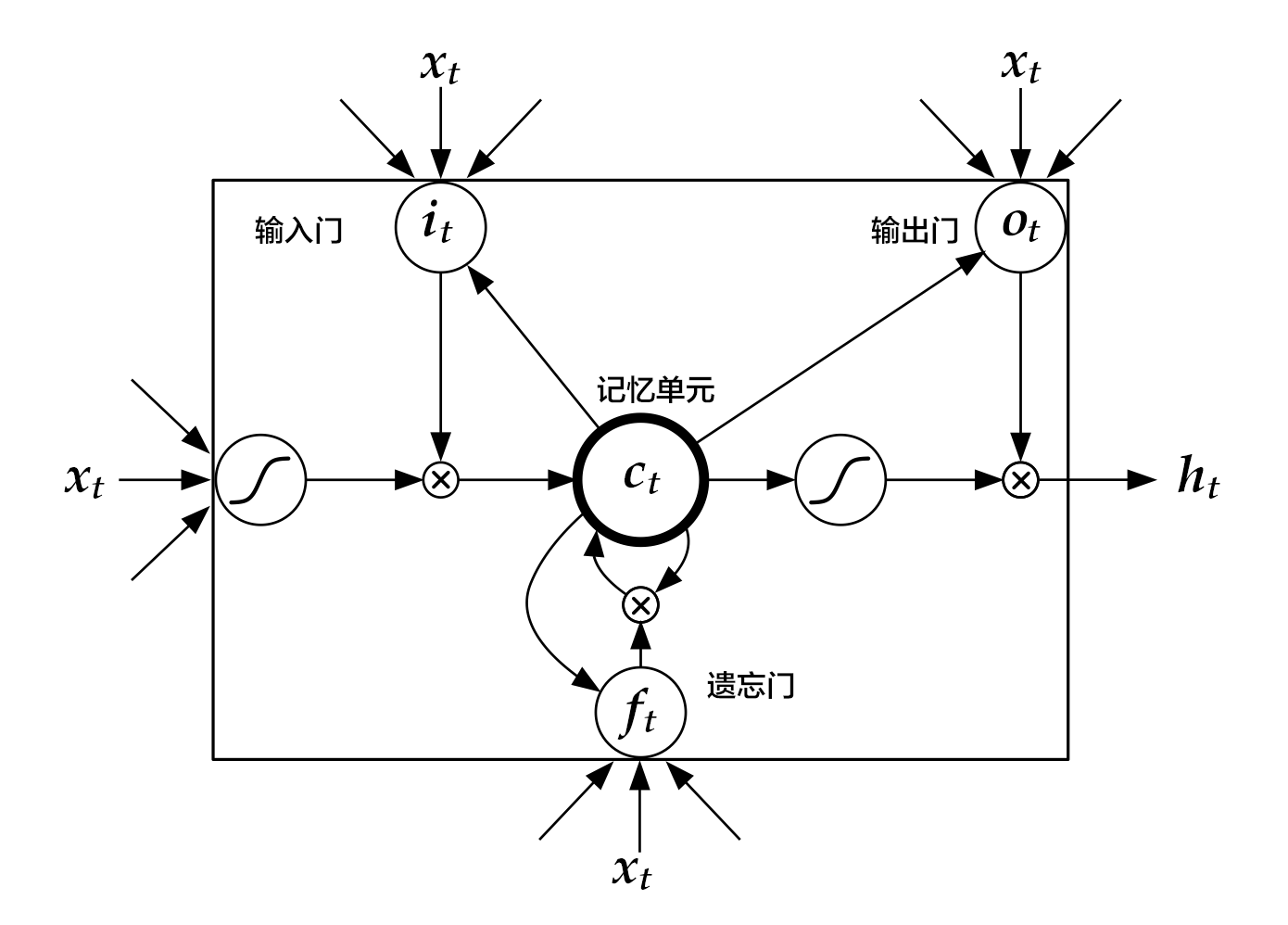- Python 2.7
- Ubuntu 16.04

情感分析

背景介绍

$\text{(#参考文献)}$
。 ## 模型概览 本章所使用的文本表示模型为卷积神经网络（Convolutional Neural Networks）和循环神经网络(Recurrent Neural Networks)及其扩展。下面依次介绍这几个模型。 ### 文本卷积神经网络简介（CNN） 我们在[推荐系统](https://github.com/PaddlePaddle/book/tree/develop/05.recommender_system)一节介绍过应用于文本数据的卷积神经网络模型的计算过程，这里进行一个简单的回顾。 对卷积神经网络来说: - 首先使用卷积处理输入的词向量序列，产生一个特征图（feature map）， - 对特征图采用时间维度上的最大池化（max pooling over time）操作得到此卷积核对应的整句话的特征， - 最后，将所有卷积核得到的特征拼接起来即为文本的定长向量表示，对于文本分类问题，将其连接至 softmax 即构建出完整的模型。 在实际应用中，我们会使用多个卷积核来处理句子，窗口大小相同的卷积核堆叠起来形成一个矩阵，这样可以更高效的完成运算。另外，我们也可使用窗口大小不同的卷积核来处理句子，[推荐系统](https://github.com/PaddlePaddle/book/tree/develop/05.recommender_system)一节的图3作为示意画了四个卷积核，不同颜色表示不同大小的卷积核操作。 对于一般的短文本分类问题，上文所述的简单的文本卷积网络即可达到很高的正确率
$\text{(#参考文献)}$
。若想得到更抽象更高级的文本特征表示，可以构建深层文本卷积神经网络
$\text{(#参考文献),(#参考文献)}$

循环神经网络（RNN）${h}_{t}=f\left({x}_{t},{h}_{t-1}\right)=\sigma \left({W}_{xh}{x}_{t}+{W}_{hh}{h}_{t-1}+{b}_{h}\right)$

长短期记忆网络（ LSTM ）

${h}_{t}=F\left({x}_{t},{h}_{t-1}\right)$

$F$$F$由下列公式组合而成：

$\begin{array}{}\text{(51)}& {i}_{t}& =\sigma \left({W}_{xi}{x}_{t}+{W}_{hi}{h}_{t-1}+{W}_{ci}{c}_{t-1}+{b}_{i}\right)\text{(52)}& \text{(53)}& {f}_{t}& =\sigma \left({W}_{xf}{x}_{t}+{W}_{hf}{h}_{t-1}+{W}_{cf}{c}_{t-1}+{b}_{f}\right)\text{(54)}& \text{(55)}& {c}_{t}& ={f}_{t}\odot {c}_{t-1}+{i}_{t}\odot tanh\left({W}_{xc}{x}_{t}+{W}_{hc}{h}_{t-1}+{b}_{c}\right)\text{(56)}& \text{(57)}& {o}_{t}& =\sigma \left({W}_{xo}{x}_{t}+{W}_{ho}{h}_{t-1}+{W}_{co}{c}_{t}+{b}_{o}\right)\text{(58)}& \text{(59)}& {h}_{t}& ={o}_{t}\odot tanh\left({c}_{t}\right)\text{(60)}& \end{array}$LSTM 通过给简单的循环神经网络增加记忆及控制门的方式，增强了其处理远距离依赖问题的能力。类似原理的改进还有 Gated Recurrent Unit (GRU) ，其设计更为简洁一些。这些改进虽然各有不同，但是它们的宏观描述却与简单的循环神经网络一样（如图2所示），即隐状态依据当前输入及前一时刻的隐状态来改变，不断地循环这一过程直至输入处理完毕：

${h}_{t}=Recrurent\left({x}_{t},{h}_{t-1}\right)$

栈式双向 LSTM （Stacked Bidirectional LSTM ）示例程序

数据集介绍

aclImdb
|- test
|-- neg
|-- pos
|- train
|-- neg
|-- pos

Paddle在dataset/imdb.py中提实现了 imdb 数据集的自动下载和读取，并提供了读取字典、训练数据、测试数据等 API。

import sys
import paddle.v2 as paddle

配置模型

文本卷积神经网络

def convolution_net(input_dim,
class_dim=2,
emb_dim=128,
hid_dim=128,
is_predict=False):
input=emb, context_len=3, hidden_size=hid_dim)
input=emb, context_len=4, hidden_size=hid_dim)
size=class_dim,
if not is_predict:
return cost
else:
return output

栈式双向 LSTM

def stacked_ LSTM _net(input_dim,
class_dim=2,
emb_dim=128,
hid_dim=512,
stacked_num=3,
is_predict=False):
"""
A Wrapper for sentiment classification task.
This network uses bi-directional recurrent network,
consisting three  LSTM  layers. This configure is referred to
the paper as following url, but use fewer layrs.
http://www.aclweb.org/anthology/P15-1109

input_dim: here is word dictionary dimension.
class_dim: number of categories.
emb_dim: dimension of word embedding.
hid_dim: dimension of hidden layer.
stacked_num: number of stacked  LSTM -hidden layer.
"""
assert stacked_num % 2 == 1

para_attr = [fc_para_attr,  LSTM _para_attr]

size=hid_dim,
act=linear,
bias_attr=bias_attr)
LSTM 1 = paddle.layer. LSTM emory(
input=fc1, act=relu, bias_attr=bias_attr)

inputs = [fc1,  LSTM 1]
for i in range(2, stacked_num + 1):
size=hid_dim,
act=linear,
param_attr=para_attr,
bias_attr=bias_attr)
input=fc,
reverse=(i % 2) == 0,
act=relu,
bias_attr=bias_attr)
inputs = [fc,  LSTM ]

size=class_dim,
bias_attr=bias_attr,
param_attr=para_attr)

if not is_predict:
return cost
else:
return output

训练模型

if __name__ == '__main__':
# init
paddle.init(use_gpu=False)

训练数据

    print 'load dictionary...'
dict_dim = len(word_dict)
class_dim = 2

    train_reader = paddle.batch(
batch_size=100)
batch_size=100)

    feeding={'word': 0, 'label': 1}

feeding用来指定train_readertest_reader返回的数据与模型配置中 data_layer 的对应关系。这里表示 reader 返回的第 0 列数据对应word层，第1列数据对应label层。

构造模型

    # Please choose the way to build the network
# by uncommenting the corresponding line.
cost = convolution_net(dict_dim, class_dim=class_dim)
# cost = stacked_ LSTM _net(dict_dim, class_dim=class_dim, stacked_num=3)

网络参数

    # create parameters
parameters = paddle.parameters.create(cost)

优化算法

    # create optimizer
learning_rate=2e-3,
model_average=paddle.optimizer.ModelAverage(average_window=0.5))

训练

    # End batch and end pass event handler
def event_handler(event):
if event.batch_id % 100 == 0:
print "\nPass %d, Batch %d, Cost %f, %s" % (
event.pass_id, event.batch_id, event.cost, event.metrics)
else:
sys.stdout.write('.')
sys.stdout.flush()
with open('./params_pass_%d.tar' % event.pass_id, 'w') as f:
trainer.save_parameter_to_tar(f)

print "\nTest with Pass %d, %s" % (event.pass_id, result.metrics)

    from paddle.v2.plot import Ploter

train_title = "Train cost"
cost_ploter = Ploter(train_title)
step = 0
def event_handler_plot(event):
global step
cost_ploter.append(train_title, step, event.cost)
cost_ploter.plot()
step += 1

    # create trainer
parameters=parameters,

trainer.train(
event_handler=event_handler,
feeding=feeding,
num_passes=2)

Pass 0, Batch 0, Cost 0.693721, {'classification_error_evaluator': 0.5546875}
...................................................................................................
Pass 0, Batch 100, Cost 0.294321, {'classification_error_evaluator': 0.1015625}
...............................................................................................
Test with Pass 0, {'classification_error_evaluator': 0.11432000249624252}

应用模型

    import numpy as np

# Movie Reviews, from imdb test
reviews = [
'Read the book, forget the movie!',
'This is a great movie.'
]
reviews = [c.split() for c in reviews]

UNK = word_dict['<unk>']
input = []
for c in reviews:
input.append([[word_dict.get(words, UNK) for words in c]])

# 0 stands for positive sample, 1 stands for negative sample
label = {0:'pos', 1:'neg'}
# Use the network used by trainer
out = convolution_net(dict_dim, class_dim=class_dim, is_predict=True)
# out = stacked_ LSTM _net(dict_dim, class_dim=class_dim, stacked_num=3, is_predict=True)

labs = np.argsort(-probs)
for idx, lab in enumerate(labs):
print idx, "predicting probability is", probs[idx], "label is", label[lab]

参考文献

1. Kim Y. Convolutional neural networks for sentence classification[J]. arXiv preprint arXiv:1408.5882, 2014.
2. Kalchbrenner N, Grefenstette E, Blunsom P. A convolutional neural network for modelling sentences[J]. arXiv preprint arXiv:1404.2188, 2014.
3. Yann N. Dauphin, et al. Language Modeling with Gated Convolutional Networks[J] arXiv preprint arXiv:1612.08083, 2016.
4. Siegelmann H T, Sontag E D. On the computational power of neural nets[C]//Proceedings of the fifth annual workshop on Computational learning theory. ACM, 1992: 440-449.
5. Hochreiter S, Schmidhuber J. Long short-term memory[J]. Neural computation, 1997, 9(8): 1735-1780.
6. Bengio Y, Simard P, Frasconi P. Learning long-term dependencies with gradient descent is difficult[J]. IEEE transactions on neural networks, 1994, 5(2): 157-166.
7. Graves A. Generating sequences with recurrent neural networks[J]. arXiv preprint arXiv:1308.0850, 2013.
8. Cho K, Van Merriënboer B, Gulcehre C, et al. Learning phrase representations using RNN encoder-decoder for statistical machine translation[J]. arXiv preprint arXiv:1406.1078, 2014.
9. Zhou J, Xu W. End-to-end learning of semantic role labeling using recurrent neural networks[C]//Proceedings of the Annual Meeting of the Association for Computational Linguistics. 2015.